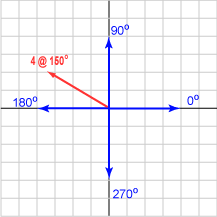A vector has length 4 and orientation 150°. Represent the vector as a column matrix

The 2D matrix is ( -3.464, 2.0 )T .The steps in this calculation are:

1. Draw a sketch: see diagram
2. Calculate x by projecting the length onto the x-axis:
4 * cos( 150 ) = -3.464
3. Calculate y by projecting the length onto the y-axis:
4 * sin( 150 ) = 2.0
4. Check answers against the sketch: Looks OK.

Be cautious about plugging into these formulae and expecting correct answers, especially when programming in C or Java. Math libraries for a programming language can do unexpected things if you are not careful.

There are three places to be especially cautious:

1. The argument for sin(), cos(), tan() is expected in radians. The return value of atan() is in radians.
2. The argument for most math functions is expected to be a double. In C, if you supply a float or an int, you won't get a error message, just a horribly incorrect answer.
3. There are several versions of "arc tan" in most C libraries, each for a different range of output values.

Now would be a good time think about radians. Usually in professional circles, angles are expressed in radians. Angles are measured counter-clockwise from the positive x axis (or sometimes a negative angle is measured clockwise from the positive x axis.

• There are 2 pi radians per full circle.
• So: 2 pi radians = 360°

### QUESTION 8:

Say that you have the `length` and `angle` of a vector. Fill in the blanks so that `vector` gets the x component and `vector` gets the y component of the vector.

```#include <math.h>

double length, angle;  /* length and angle of a vector */
double vector;      /* elements of the vector */

. . .

length = some value
angle  = some number of degrees

vector =

vector =
```

(Assume that the symbol for pi is `M_PI`. Unfortunately, different compilers use different symbols for PI and define it in different header files. You should use the symbol appropriate for your system rather than define PI on your own.)

(If you don't know C, just pretend this is Java.)